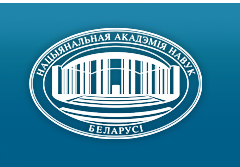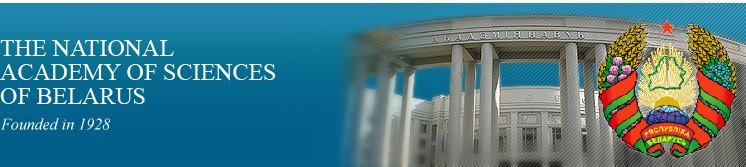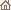Home News About Academy Academy Activities Academy Membership Organizations Publications Conferences Contacts Site Map

# Proc. of the Natl. Academy of Sciences of Belarus, Ser. Phys.-Math. Sci., 2002, No.2/ Publications / Scientific Journals

Proc. of the Natl. Academy of Sciences of Belarus, Ser. Phys.-Math. Sci., 2002, No.2Proceedings of the National Academy of Sciences of Belarus (Vestsi Natsiyanalnai Akademii Navuk Belarusi) SERIES OF PHYSICAL-MATHEMATICAL SCIENCESPublished by The Institute of Mathematics of NASB, Minsk, Republic of Belarus

### Number 2, 2002## CONTENTS

### MATHEMATICS

Kostyukova O. I.
Path-following method for a family of semi-infinite programming problems. pp. 5--10

Summary: A one-parametric family of convex semi-infinite problems depending on a parameter t Î [0, t*] is considered. For fixed t = t0 Î [0, t*] rules of constructing the solutions of this family for t in a right-side neighborhood of t0 are described. Results on one-sided derivatives of solution with respect to the parameter are presented. On the basis of the obtained results an active-set-strategy and a path-following algorithm are suggested.

Zhuk E. E., Avramenko N. A.
Cluster analysis of autoregression time series in a space of ML-estimators of autoregression parameters. pp. 11--16

Summary: The problem of cluster analysis of observations described by the autoregression time series is considered. The clustering procedure in a space of ML-estimators of autoregression parameters is proposed and its efficiency is analytically and experimentally investigated.

Zalesskii B. A.
Bayesian estimations of grayscale images by use of maximum network flow algorithms. pp. 17--22

Summary: Two Bayesian models of gray-scale images are described. The maximum-a-posteriori estimates of these models can be efficiently identified by parallel use of the maximum network flow methods.

Malyutin V. B.
Approximations of functional integrals with the Clifford values. pp. 23--25

Summary: Functional integral with noncommuting Clifford values is approximated by functional integral where noncommuting values axe separated. It allowes to overcome the noncommuting values problem

Petrov V. A.
Approximations functional integrals on some Gaussian measures. pp. 26--29

Summary: Composite approximate formulas for evaluation some Gaussian functional integrals are constructed. The estimates of remainders of these approximations are obtained.

Rusak V. N. Tolmasheva M. S.
Hermite-type interpolation and quadrature formulas which are exact for rational functions. pp. 30--33

Summary: Gauss-type quadrature formulas for rational functions with simple poles has been investigated.

Tykun A. S.
On the the Conley index evaluation for 3D dynamical systems. pp. 34--38

Summary: The 3D dynamical system without singular points is considered. The existence of the bounded solution is proved using the Conley index. For an arbitrary compact area in three dimensional space the sufficient condition of being an isolating area is proved.

Galmak A. M.
Manyfolds of polyadic groups. pp. 39--40

Summary: The varieties of n-axy groups of the M*(n) form are defined and considered, where M is variety of groups.

Hodalevich A. D.
Projectors of soluble universal algebras. pp. 41--43

Summary: The following result is proved. Let A be a finite soluble universal algebra containing some one-elements subalgebras, then A has at least one F-projector for any non-empty saturated formation F Í m.

Benediktovitch I. I.
About the structure of the Specht's module. pp. 44--45

Summary: The set of all homogeneous products of commutators in the free associative algebra over field of characteristic 0 is called a Specht's module. It is a natural module over the symmetric group Sn. We have obtained its structure as Sn-module. Namely, it is isomorphic to the sum Sni=2 (-1)i[1i] ­ n where [1i] is an Young table corresponding to the one dimensional alternating representation and [1i] ­ n denotes a module induced from Si to Sn.

Silaeva N. A.
Simultaneous approximations of zero by values of normal curves in different metrics. pp. 46--52

Summary: The extremality of plane curves with positive curvature in the space R x C x Zp is proved.

Sarazhinskii D. S.
Invariant cones and eigenvectors for the Perron--Frobenius operator in spaces of the Holder functions. pp. 53--56

Summary: The strong invariant cone for the Perron--Frobenius linear operator generated by the shift mapping on a space of sequences is constructed. Existence of the cone was allowed to define ergodic invariant measures on the space of sequences, to prove probability limit theorems and to obtain presize asymptotics for large deviations.

Akinfina M. A.
Estimation of a spectrum of a discrete stationary symmetric a-stable process in case of using Bartlett's periodogram. pp. 57--60

Summary: A consistent estimate of spectral density of a symmetric stationary a-stable casual process is being designed in case of using Bartlett's periodogram. Its convergence in probability to spectral density is established. The rate of convergence of the considered estimate is investigated in case of using polynomial kernel Jekson's type and depending on a smoothness of a spectral density.

### PHYSICS

Bogush A. A., Kisel V. V., Tokarevskaya N. G., Red'kov V. M.
Nonrelativistic limit in generally covariant theory of a vector particle. pp. 61--66

Summary: The known procedure of obtaining the non-relativistic limit in the Duffin -- Kemmer theory of a vector particle in flat Minkowski space-time is generalized to a possible non-Euclidean space-time geometry. It is shown that the generally covariant Duffin -- Kemmer equation permits such a limit if a metric tensor satisfies the relations g00(x) = 1, g0i(x) = 0. In the frames of the Tetrode -- Weyl -- Fock -- Ivanenko tetrad formalism a generally covariant equation for 3-component wave function describing the vector particle is produced. It is correct in presence of an external electromagnetic field and of a curved 3-space background with the metric of the form dS2 = (dx0)2 - gij(x0, xk)dxidxj.

Pilipovich V. A., Esman A.K., Goncharenko I. A., Kuleshov V. K., Sergienya S. A.
Dynamic of the generation frequency tuning of single-frequency fibre and waveguide lasers with controllable Bragg grating. pp. 67--71

Summary: The dynamic of radiation wavelength tuning of fibre and waveguide lasers by the use of Bragg grating on the base of waveguide electrooptical modulator is analysed. Dependence of the switching time on the power of signal remaining on the wavelength into which the radiation is tuned, on concentration of doping elements, reflectivity of cavity output mirror and optical pump power is estimated. It is shown that lasers with output mirror reflectivity around 95% possess the minimal tuning time and maximal generation efficiency. In lasers with cavity length 1 cm and optimal characteristics the time of switching to another wavelength does not exceed 0.7 ns in all region of spectral tuning.

Kudryashov V. V., Vanne Yu. V.
Quasi-uniform approximation for power-law potentials. pp. 72--76

Summary: Analytical solutions of the Schrodinger equation with power-law potentials are obtained in the framework of the new quasi-uniform approximation based on explicit summation of the constituent WKB series. The detailed numerical verification of the proposed method is performed.

Kurilkina S. N., Shuba M. V.
Bistability of acoustooptic interaction in gyrotropic crystals with electroinduced anisotropy. pp. 77--81

Summary: It has been shown that conditions and peculiarities of bistable regimes by acoustoelectrooptic interaction in cubic non-centrosymmetrical crystals are determined by presence of gyrotropy. It has been established that more essentially the latter is displaying i doubling of number of bistable regions and in appearance of dependence of conditions for realization of bistable regimes on polarization of incident light wave. It has been obtained the opportunity of change of switching character by reversion of external electric field.

Makovetskii G. I., Galyas A. I., Demidenko O. F.
Obtaining and magnetic properties of Mn1-xTixSe (0 £ x £ 0.2) and Mn1-xVxSe (0 £ x £ 0.15) solid solutions. pp. 82--84

Summary: Under the high pressure and the high temperature conditions theMn1-xTixSe (0 £ x £ 0.2) and Mn1-xVxSe (0 £ x £ 0.15) solid solutions were synthesized. All samples have the cubic lattice like MnSe. The magnetic susceptibility for all the investigated samples was determined in temperature interval 77 -- 900 K. The antiferromagnetic ordering have been observed for all the solid solutions at T < 130 -- 150 K.

Akimov A. I., Savchuk G. K., Rubzov V. A., Letko A. K.
Research of influence of cationic and anionic deficiency on the crystal structure of lead titanate. pp. 85--90

Summary: Usung by the Rietveld method parameters of crystal structure of lead titanate were refined in depending on a degree anionic and cationic defectiviti. X-Ray difractometer DRON-4 (CuKa, 20° < 2q < 70°, step 0.01°, exposition in a point -- ten sec.) was used. All accounts are carried out by using the program "GSAS" in the framework of space group P4mm. The refinement of parameters of structure is carried out in isotropic approximation Rp 5.0 -- 6.0.

Gusakov V. E.
On anomalous temperature behaviour of HTSC compounds elastic properties. pp. 91--96

Summary: It was established that experimentally observed anomalous (hysteretic) temperature dependencies of elastic properties of YBa2Cu3O7-d crystals are related to the hysteretic behavior of the elastic modulus C3333 and are caused temperature-dependent renormalization of force constants of O(4) (apex) oxygen atoms with copper atoms.

Nupriyonok I. S., Shibko A. N.
Phase changes of composition Zr -- Si under UV-irradiation. pp. 97--101

Summary: Phase transformations of thin films Zr and composition of Zr -- Si under vacuum stacionary annealing with simultaneous UV-irradiation have been investigated. Phase changes, change of the boundary metal -- semiconductor cause change in contact electrophysical characteristics. The simultaneous treatment of the Zr -- Si compositionmakes it possible to form a rectifying contact with given parametes depending on light irradiation power.

Brinkevich D. I., Lukashevich M. G., Petrov V. V., Prosolovich V. S., Skripka D. A., Yankovskii Yu. N.
Giant magnitoresistance in rare-earth doped silicon. pp. 102--104

Summary: Negative magnitoresistance at liquid nitrogen temperature was found in silicon doped by rare-earth elemets Er, Gd and Ho at the concentrations 3 • 1015 -- 2.3 • 1017 cm-3. It cause by giant magnitoresistance consist of rare-earth clusters magnetic moments orientation by external magnetic field that charge carriers scattering decrease.

### INFORMATICS

Tikhonenko O. M.
The analysis of processor sharing queueing system with non-homogeneous demands. pp. 105--111

Summary: The sum tags vector characteristics for M/G/1/¥ and M/G/1/m processor sharing queues are determined taking into account the dependence between tags values and service time of the demand. The stationary probability of demand's losing is estimated for the case of restricted summarized volume.

Naidenko V. G.
The complexity of finding a second solution to a NP-complete problem. pp. 112--113

Summary: The NP-complete problem is constructed. Every instance of the problem contains either no solution or at least two solutions. There is no polynomial-time algorithm which for given example of the problem and its solution could find the second solution with the following condition NP Ç co-NP ¹ P.

Zaika V. A., Vankovich G. R., Lopareva N. V., Povarov A. A.
Method of information "section" in study of electromagnetic complex technical system compatibility. pp. 114--118

Summary: The method of constraction of the information "sections" of multichannel data, which make it possible to decrease the number of measuring channels under the carrying out of the electromagnetic compatibility tests of the complex technical systems is proposed and analysed.

Bakhanovich S. V.
The synthesis of onedimensional scheduling function generated by the multidimensional scheduling. pp. 119--122

Summary: The method of onedimensional scheduling function synthesis is presented. The method is considered in the frameworks of multidimensional scheduling and space-time mapping of n-dimensional algorithms with uniform dependences onto (n - r-dimensional parallel architectures.

### ABSTRACTS OF DEPOSITED PAPERS

Shishkevich A. A.
Finite represented semi aspherical pro-p-groups. p. 123

Pavlov L. I.
Photodiod electric optical measuring instrument for the lengthy micro objects. p. 123/ Publications / Scientific Journals / Back to Top of Page

Designed and maintained by Dr. Nikolai N. Kostyukovich. Last updated: October 8, 2002
Created with assistance of Dr. Ignatii I. Korsak
Copyright © 2002 The National Academy of Sciences of Belarus
Copyright © 2002 The Institute of Mathematics of NASB